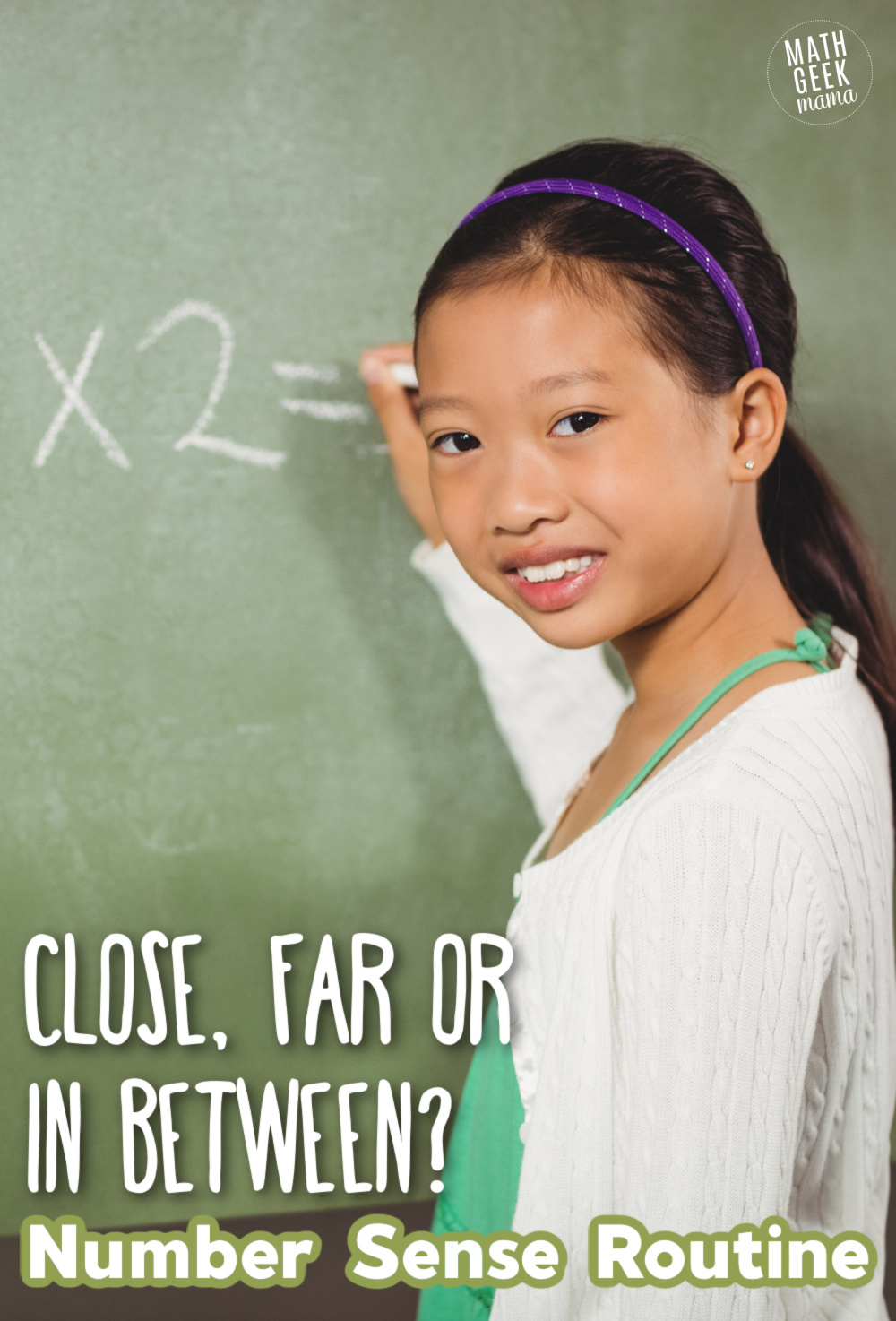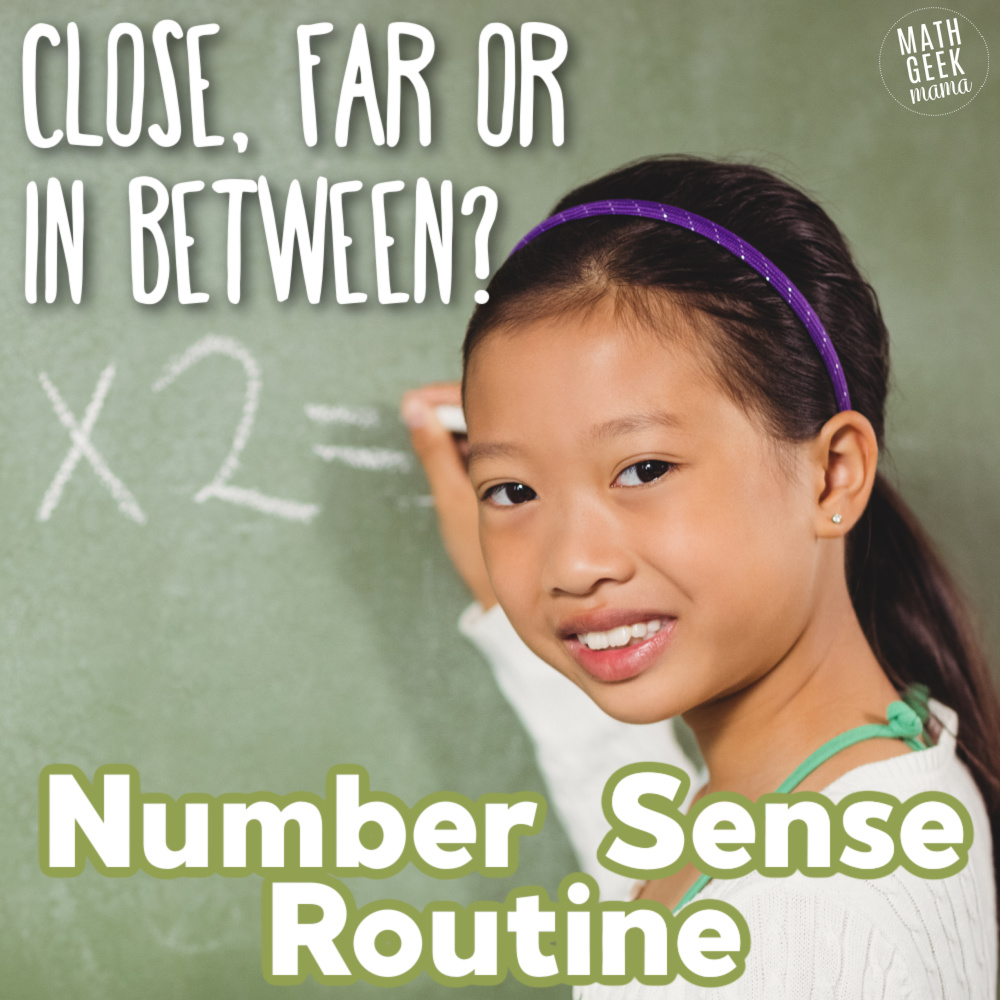# Simple Yet Powerful Math Number Sense Routine: Close, Far, In-Between

Looking for an easy way to weave daily number sense practice into your math routine? Learn what number sense routines are & get started with this simple number sense routine known as close, far or in-between.

Mathematics is often perceived as a daunting subject, filled with complex equations and abstract concepts. However, at its core, math is about understanding relationships, patterns, and making sense of the world around us. Number sense routines are a powerful tool for developing a deep and intuitive understanding of numbers, paving the way for mathematical success. In this blog post, we’ll explore what number sense routines are, why they matter, how they can benefit learners of all ages, and a freebie for one of my favorites: Close, Far, or In-Between Number Sense Routine. Read even more about the Close, Far, or In-Between Number Sense Routine HERE.*This is a guest post from Danielle at Math MindED Teaching

## What Are Number Sense Routines?

Number sense routines are short, structured activities designed to help individuals develop an intuitive understanding of numbers and their relationships.

These routines focus on fundamental mathematical concepts, such as counting, comparing, and estimating, and can be adapted for learners of all ages, from early childhood to adulthood.

## Why Are Number Sense Routines Important?

1. Build a Strong Foundation: Number sense is the foundation upon which more advanced mathematical concepts are built. Developing a strong number sense early in one’s mathematical journey can lead to better comprehension of higher-level math.
2. Enhance Problem-Solving Skills: Number sense routines promote critical thinking and problem-solving abilities. When individuals have a deep understanding of numbers, they can tackle complex problems more effectively.
3. Foster Mathematical Confidence: Math anxiety is a common issue among learners of all ages. Number sense routines provide a low-stress, enjoyable way to engage with math, helping individuals build confidence in their abilities.
4. Real-Life Application: Number sense is not just confined to the classroom. It’s a skill that’s highly relevant in everyday life, from budgeting and cooking to making informed decisions based on data.
5. Adaptability: Number sense routines can be tailored to suit the needs and abilities of learners at different levels, making them an excellent tool for both educators and self-learners.

## Examples of Number Sense Routines

1. Which One Doesn’t Belong? – Present a set of numbers or shapes and ask learners to identify which one doesn’t belong and explain their reasoning.
2. Estimation Jar – Fill a jar with objects (e.g., marbles, beans) and ask learners to estimate how many are in the jar. Then, count to check their estimates.
3. Number Talks – Engage in open-ended discussions about mathematical problems, encouraging learners to share their strategies and solutions.
4. Number Strings –  A set of related math problems designed to teach strategies based on number relationships. It is a 10-15 minute routine that can be used for any math topic.
5. Counting Collections – Gather a collection of objects and have learners count them in various ways (e.g., by twos, by fives, by tens).
6. Counting Circles – Students stand and form a circle facing in toward each other. The teacher selects a counting sequence to practice. Children take turns counting a number one at a time around the circle. Count by whole numbers, fractions, or decimals!
7. Choral Counting – Activity in which the teacher leads children in counting aloud together by a given number. As the class calls out each number the teacher records the count on the board, pausing the count at strategic moments and recording patterns. Again, you can count by whole numbers, fractions, or decimals!
8. Mental Math Challenges – Pose simple addition, subtraction, multiplication, or division problems for learners to solve mentally within a time limit.
9. Same or Different? –  A routine that provides students opportunities to construct arguments when comparing objects, such as numbers or shapes. Pictures can be strategically created to elicit strategy related mathematical discourse.
10. Two Truths, One Lie – Students are presented with three facts, images, or statements (only two of which are true). The objective is for students to identify which statement is false and justify why.
11. Missing Midpoint/Missing Endpoint – This routine involves a number line with two endpoints and a missing midpoint. This routine encourages students to find the midpoint by thinking about dividing the number line into equal parts.
12. Close, Far, or In-Between – This activity focuses on number sense development and relative magnitude. When thinking about relative magnitude, students can compare and refer to the size relationship one number has with another.

## Close, Far, or In-Between Number Sense Routine

For this routine, the teacher displays three numbers for students to review.

• For kindergarten students, use smaller numbers (0-10).
• For first and second graders, use 2 digit numbers.
• For third grade students, use 3 digit numbers or simple fractions.
• For fourth and fifth grade students use large numbers, fractions and decimals.
• For an extra challenge or for middle school students use positive/negative integers.

Then ask questions that encourage relative magnitude relations. Be sure to encourage mathematical discourse.

Some examples of questions to pose to your students:

• Which two are closest together?
• Which number is closest to 10? 50? 100? 500? etc.
• Name a number that is greater/less than all three
• Name a number (or fraction/decimal) between __ & __ (2 of the numbers)
• How far apart are __ & __? (2 of the numbers)

I love using Close, Far, or In-Between to teach DECIMAL understanding.

This number sense routine helps students to visually make sense of decimals on a number line.

Would you like a FREE Decimal version of the Close, Far, or In-Between Number Sense Routine? Check it out HERE.

Can’t get enough DECIMAL products for your students? Then check out these other DECIMAL resources for students in grades 4-6.Number sense routines are not just about crunching numbers; they are about developing a deep and intuitive understanding of mathematics. These routines empower learners to see math as a language that helps them make sense of the world.

By incorporating number sense routines into our educational practices and daily lives, we can cultivate mathematical intuition, enhance problem-solving skills, and build a strong foundation for lifelong learning. So, whether you’re a teacher, a parent, or a lifelong learner, embrace number sense routines as a valuable tool on your mathematical journey.

### Never Run Out of Fun Math Ideas

If you enjoyed this post, you will love being a part of the Math Geek Mama community! Each week I send an email with fun and engaging math ideas, free resources and special offers. Join 163,000+ readers as we help every child succeed and thrive in math! PLUS, receive my FREE ebook, 5 Math Games You Can Play TODAY, as my gift to you!